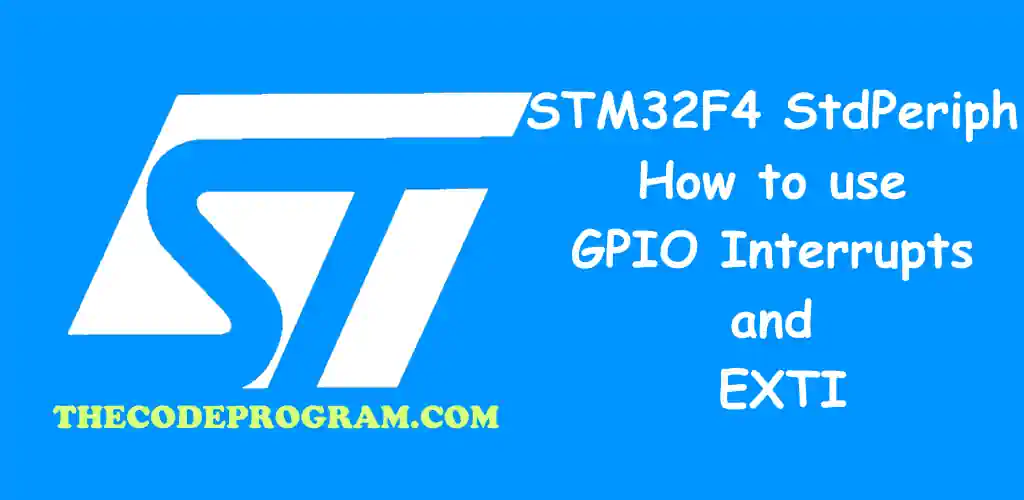## STM32F4 StdPeriph : How to use GPIO Interrupts and EXTI

Hello everyone, in this article we are going to talk about interrupt management of GPIO's with EXTI at STM32F4 StdPeriph. We use here STM32F4 Development board and built-in leds on discovery board.

Let's get started.

Firstly, What is interrupt
Interrputs are one of key functions of programming. We can see interrupt system almost every programming areas. ARM based microcontrollers have NVIC. Function of NVIC is mapping the external interrupts for the MCU. NVIC has the triggers for all interrupts.

In here we are going to External Interrupt Controller to fire a function when an input activated. It controls the inputs and when a GPIO pin has an input HIGH, EXTI fires a its handler function to perform specified operation.

Now, let's start to coding

Firstly we are going to declare a variable which I will hold the button pressed counter.
``````
int button_counter = 0;
``````

Now let's write our main function. Below code block is in our main function.

Below you can see buil-in connections.
• PA0 Blue Button
• PD12 Green LED
• PD13 Orange LED
• PD14 Red LED
• PD15 Blue LED
Firstly we are going to enable our required Periphal Bus Frequencies.
``````
RCC_AHB1PeriphClockCmd(RCC_AHB1Periph_GPIOA, ENABLE);
RCC_AHB1PeriphClockCmd(RCC_AHB1Periph_GPIOD, ENABLE);
``````
Then we enable and configure the required GPIO's here. In here we are going to enable Built-in LED pins as output and Blue Button pin as input.
``````
GPIO_InitTypeDef   GPIO_InitStructure;

GPIO_InitStructure.GPIO_Mode = GPIO_Mode_OUT;
GPIO_InitStructure.GPIO_Pin = GPIO_Pin_12 | GPIO_Pin_13 | GPIO_Pin_14 | GPIO_Pin_15;
GPIO_InitStructure.GPIO_OType = GPIO_OType_PP;
GPIO_InitStructure.GPIO_Speed = GPIO_Speed_100MHz;
GPIO_Init(GPIOD, &GPIO_InitStructure);

GPIO_InitStructure.GPIO_Mode = GPIO_Mode_IN;
GPIO_InitStructure.GPIO_PuPd = GPIO_PuPd_NOPULL;
GPIO_InitStructure.GPIO_Pin = GPIO_Pin_0;
GPIO_Init(GPIOA, &GPIO_InitStructure);
``````
Now we are going to enable our EXTI. Pin0 is connected to EXTI_Line0, so we have activated the EXTI_Line0.
``````
EXTI_InitTypeDef   EXTI_InitStructure;
EXTI_InitStructure.EXTI_Line = EXTI_Line0;
EXTI_InitStructure.EXTI_Mode = EXTI_Mode_Interrupt;
EXTI_InitStructure.EXTI_Trigger = EXTI_Trigger_Rising;
EXTI_InitStructure.EXTI_LineCmd = ENABLE;
EXTI_Init(&EXTI_InitStructure);
``````
Now we need to enable NVIC. This NVIC will control the EXTI which we declared above so our pin.
``````
NVIC_InitTypeDef   NVIC_InitStructure;
NVIC_InitStructure.NVIC_IRQChannel = EXTI0_IRQn;
NVIC_InitStructure.NVIC_IRQChannelPreemptionPriority = 0x0F;
NVIC_InitStructure.NVIC_IRQChannelSubPriority = 0x0F;
NVIC_InitStructure.NVIC_IRQChannelCmd = ENABLE;
NVIC_Init(&NVIC_InitStructure);
``````

Until here our main function is ready. Now we are going to declare interrupt handler function.

Below code block you can see the interrupt handler function. This interrupt handler function will enable one of led at every pressing the blue button.
``````
void EXTI0_IRQHandler(void)
{
if(EXTI_GetITStatus(EXTI_Line0) == SET)
{
button_counter++;
if(button_counter %4 == 0){
GPIO_SetBits(GPIOD,GPIO_Pin_12);
GPIO_ResetBits(GPIOD,GPIO_Pin_13 | GPIO_Pin_14 | GPIO_Pin_15);
}
else if(button_counter %4 == 1){
GPIO_SetBits(GPIOD,GPIO_Pin_13);
GPIO_ResetBits(GPIOD,GPIO_Pin_12 | GPIO_Pin_14 | GPIO_Pin_15);
}
else if(button_counter %4 == 2){
GPIO_SetBits(GPIOD,GPIO_Pin_14);
GPIO_ResetBits(GPIOD,GPIO_Pin_12 | GPIO_Pin_13 | GPIO_Pin_15);
}
else if(button_counter %4 == 3){
GPIO_SetBits(GPIOD,GPIO_Pin_15);
GPIO_ResetBits(GPIOD,GPIO_Pin_12 | GPIO_Pin_13 | GPIO_Pin_14);
}

/* Clear the EXTI line 0 pending bit */
EXTI_ClearITPendingBit(EXTI_Line0);
}
}
``````

As you can see above we have never talked about a while loop. All of this operations is happening at GPIO interrupt handler.

Burak Hamdi TUFAN

05/01/2020

14/05/2020

01/11/2020